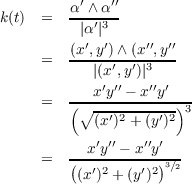## Math 501: Differential Geometry

Homework 1 <me@tylerlogic.com>

January 26, 2013
http://coursework.tylerlogic.com/courses/math501/homework01

1 do Carmo pg. 5 exercise 2

Assume for later contradiction that α(t0) is not orthogonal to α(t0). Since α(t0) is nonzero, then we are able to find a ϵ > 0 such that either |α(t0 -ϵ)| < |α(t0)| or |α(t0 + ϵ)| < |α(t0)|. However, either case contradicts the fact that α(t0) is the point of the trace of α which is closest to the origin. Thus α(t0)α(t0) must be true.

2 do Carmo pg. 7 exercise 2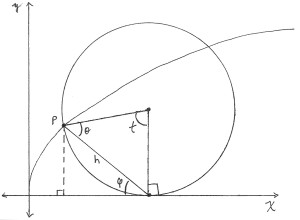Figure 1: Cycloid at a Glance.

(a)

We will parametrize the cycloid by the angle of rotation, denote it by t. From the diagram in Figure 1 it can be seen that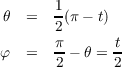Thus the value of h is determined by the following sequence of equations.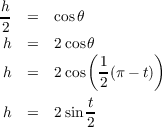With these things, we can finally derive the point p.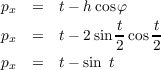and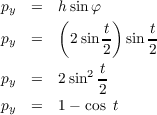(b)

Let’s rotate from 0 to 2π, fixing t0 at 0. By making use of the double angle formula for cos, we have the following.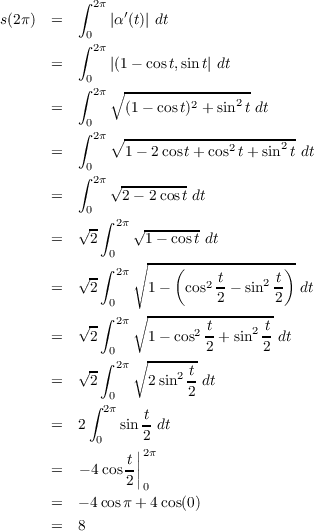3 do Carmo pg. 7 exercise 3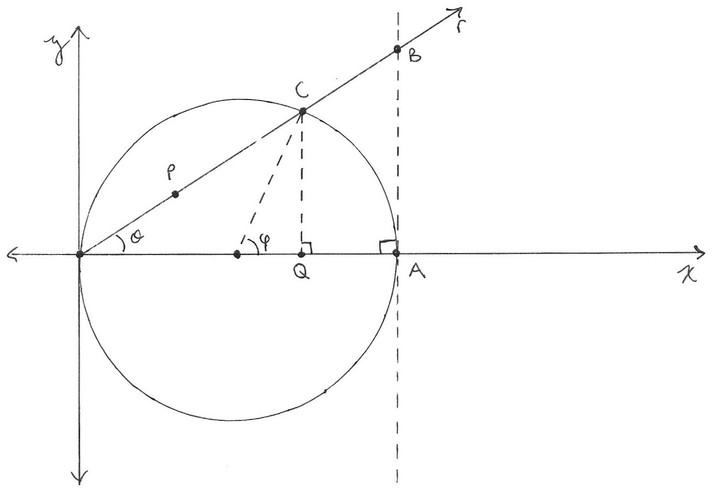Figure 2: Cissoid of Diocles at a Glance.

(a)

For this problem we will use Figure 2 as a guide. Using some trigonometry, from the figure we get the following (remember the diameter is length 2a)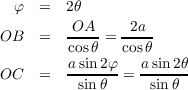These allow us to have the following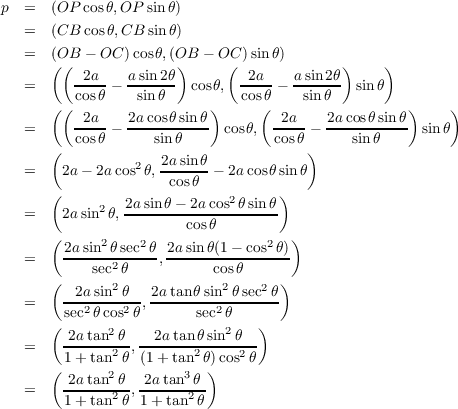Hence letting t = tanθ we get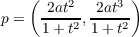(b)

Because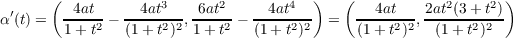then α(0) = (0,0), and so α has a singular point at the origin (0,0).

(c)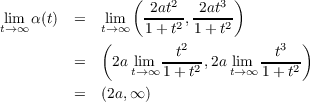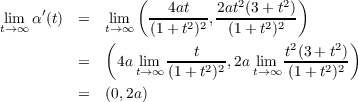4 do Carmo pg. 11 exercise 10

(a)

Left Equation For α(t) = (x(t),y(t),z(t)) the Fundamental Theorem of Calculus gives us that there is a c I such that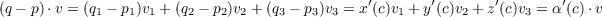but since v is a vector of magnitude 1, then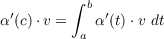so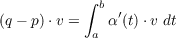Right Inequality By the definition of dot product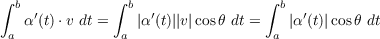Now since cosθ ranges between -1 and 1 then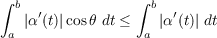(b)

5

(a)

To show that α(t) is parametrized by arc lenght, we must show |α(t)| = 1 for all t. So proceeding that way, we have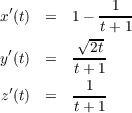giving us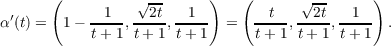Therefore |α(t)| is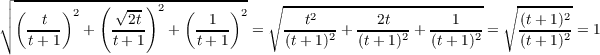Hence α is parametrized by arc length.

(b)

Curvature. The curvature, κ, is |α′′(t)|. Using what we know from the previous part,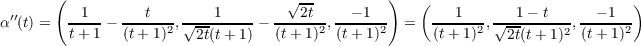then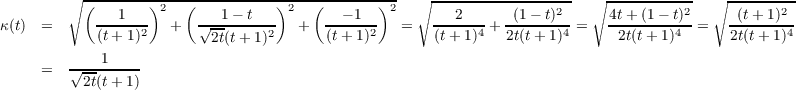Torsion. The torsion of α, τ, is the magnitude of the derivative of the binormal vector, b, as given by the Frenet equation b= τn. The binomial vector is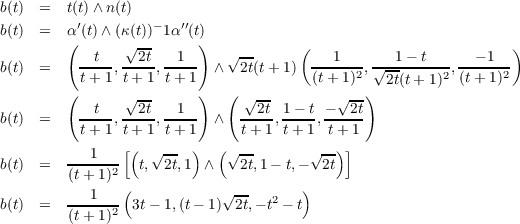so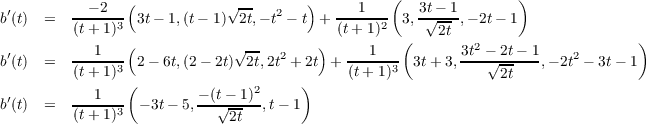and thus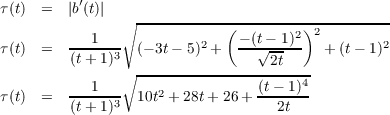6 do Carmo pg. 22 exercise 1

The following are for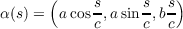(a) The parameter s is the arc length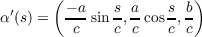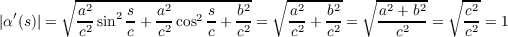Therefore s is the arc length.

(b) Curvature and torsion.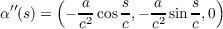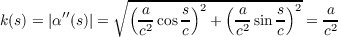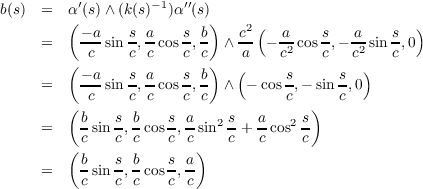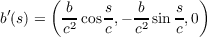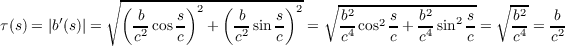(c)

The osculating plane of α at s = s0 is defined by the following point, normal vector tuple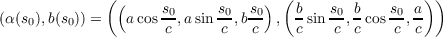the equations for which are given and determined in the previous problem.

(d)

The angle between the z-axis and a line passing through α(s) and containing n(s) will be the same as the angle between n(s) and any vector contain by the z-axis, say (0,0,1). And that angle will be the inverse cosine of their dot product, since both vectors have magnitude of one.

Since n(s)(k(s))-1α′′(s) =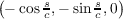, then the dot product of it and (0,0,1) is zero, implying that the cosine of the angle between them is zero, which in turn confirms that the angle between them is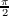.

(e)

This is similar to the previous problem except that we will use t(s) in place of n(s). Because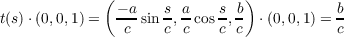i.e. the cosine of the angle between them is a constant, then the angle bewteen t and (0,0,1) is constant, since they both have a magnitude of one.

7 do Carmo pg. 25 exercise 12

(a)

Since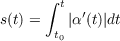then by the fundamental theorem of calculus we have ds∕dt = |α(t)|, which implies dt∕ds = 1|α(t)|.

Taking the derivative of both sides of the above equation leads to the following sequence of equations.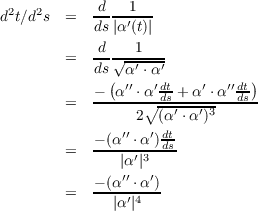(b)

(c)

(d)

Assuming that the yet-to-be-proven part (b) of this problem is true, then the signed curvature is